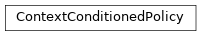# garage.torch.policies.context_conditioned_policy¶

A policy used in training meta reinforcement learning algorithms.

It is used in PEARL (Probabilistic Embeddings for Actor-Critic Reinforcement Learning). The paper on PEARL can be found at https://arxiv.org/abs/1903.08254. Code is adapted from https://github.com/katerakelly/oyster.

class ContextConditionedPolicy(latent_dim, context_encoder, policy, use_information_bottleneck, use_next_obs)

Bases: torch.nn.ModuleA policy that outputs actions based on observation and latent context.

In PEARL, policies are conditioned on current state and a latent context (adaptation data) variable Z. This inference network estimates the posterior probability of z given past transitions. It uses context information stored in the encoder to infer the probabilistic value of z and samples from a policy conditioned on z.

Parameters
• latent_dim (int) – Latent context variable dimension.

• context_encoder (garage.torch.embeddings.ContextEncoder) – Recurrent or permutation-invariant context encoder.

• policy (garage.torch.policies.Policy) – Policy used to train the network.

• use_information_bottleneck (bool) – True if latent context is not deterministic; false otherwise.

• use_next_obs (bool) – True if next observation is used in context for distinguishing tasks; false otherwise.

reset_belief(self, num_tasks=1)

Reset $$q(z \| c)$$ to the prior and sample a new z from the prior.

Parameters

sample_from_belief(self)

Sample z using distributions from current means and variances.

update_context(self, timestep)

Append single transition to the current context.

Parameters

timestep (garage._dtypes.TimeStep) – Timestep containing transition information to be added to context.

infer_posterior(self, context)

Compute $$q(z \| c)$$ as a function of input context and sample new z.

Parameters

context (torch.Tensor) – Context values, with shape $$(X, N, C)$$. X is the number of tasks. N is batch size. C is the combined size of observation, action, reward, and next observation if next observation is used in context. Otherwise, C is the combined size of observation, action, and reward.

forward(self, obs, context)

Given observations and context, get actions and probs from policy.

Parameters
• obs (torch.Tensor) –

Observation values, with shape $$(X, N, O)$$. X is the number of tasks. N is batch size. O

is the size of the flattened observation space.

• context (torch.Tensor) – Context values, with shape $$(X, N, C)$$. X is the number of tasks. N is batch size. C is the combined size of observation, action, reward, and next observation if next observation is used in context. Otherwise, C is the combined size of observation, action, and reward.

Returns

• torch.Tensor: Predicted action values.
• np.ndarray: Mean of distribution.

• np.ndarray: Log std of distribution.

• torch.Tensor: Log likelihood of distribution.

• torch.Tensor: Sampled values from distribution before

applying tanh transformation.

torch.Tensor: z values, with shape $$(N, L)$$. N is batch size.

L is the latent dimension.

Return type

tuple

get_action(self, obs)

Sample action from the policy, conditioned on the task embedding.

Parameters

obs (torch.Tensor) – Observation values, with shape $$(1, O)$$. O is the size of the flattened observation space.

Returns

Output action value, with shape $$(1, A)$$.

A is the size of the flattened action space.

dict:
• np.ndarray[float]: Mean of the distribution.

• np.ndarray[float]: Standard deviation of logarithmic values

of the distribution.

Return type

torch.Tensor

compute_kl_div(self)

Compute $$KL(q(z|c) \| p(z))$$.

Returns

$$KL(q(z|c) \| p(z))$$.

Return type

float

property networks(self)

Return context_encoder and policy.

Returns

Encoder and policy networks.

Return type

list

property context(self)

Return context.

Returns

Context values, with shape $$(X, N, C)$$.

X is the number of tasks. N is batch size. C is the combined size of observation, action, reward, and next observation if next observation is used in context. Otherwise, C is the combined size of observation, action, and reward.

Return type

torch.Tensor